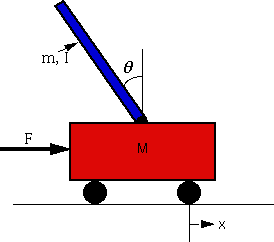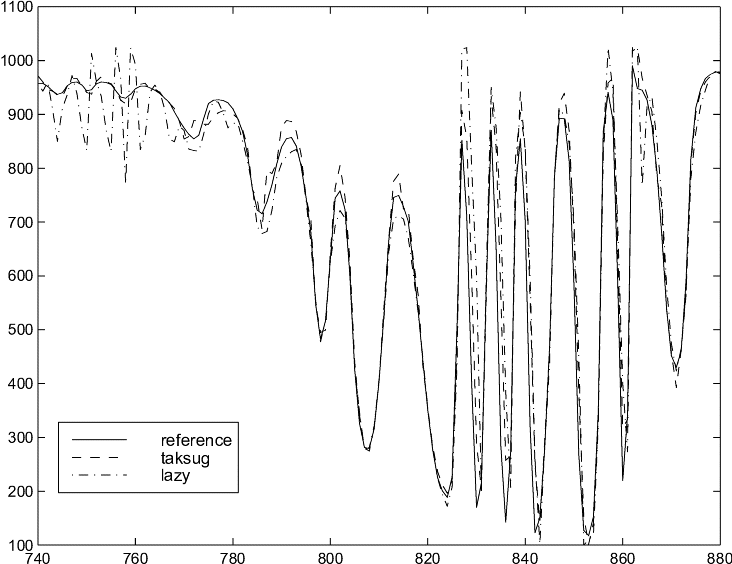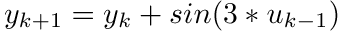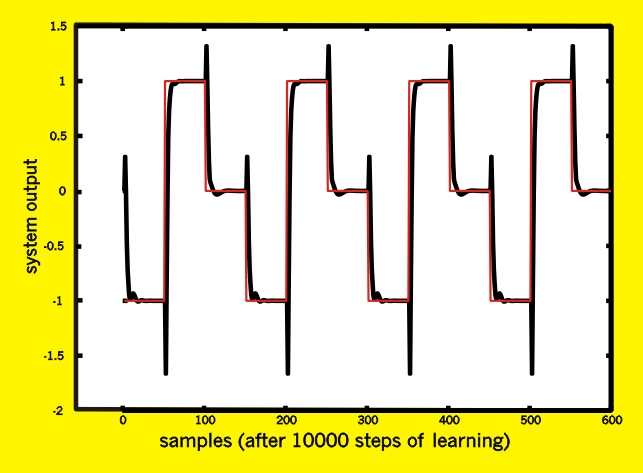# FUZZY CONTROL

In the year 2000, I worked with 2 colleagues (Antoine Duchâteau and Edy Bertolissi) on the European LTR projet FAMIMO (FAMIMO stands for "Fuzzy Algorithms for the Control of Multi-Input, Multi-Output Processes"). The main goal of this project was to see if the fuzzy control is a viable alternative to the classical PID control. Apparently the answer is no. During the project, we develloped the NLMIMO toolbox. The NLMIMO toolbox is composed of two main modules:
• an identification module using (fuzzy/ lazy learning/ classical linear) techniques.
• a multiple input, multiple ouput, multiple step ahead, direct self-adaptive fuzzy controller.

The first module has been implemented by Antoine Duchâteau. The second module has been mainly programmed by myself. The controller is from a GPC type (global predictive control). GPC controllers simulate the process to control in the future using prediction/identification techniques. Based on this simulation, we can calculate the error E between the setpoints and the real ouput of the process. This error E is a function depending on the control signals u generated by the controller. These signals u themselves depend on the parameter w of the fuzzy rules inside the controller. Thus, we will try to find the value of w which minimize the error E(u(w)). The minimization algorithm (the optimization algorithm) is a simple "steppest descent step" (see my thesis about optimization). This learining algorithm is used on-line. The controller is thus adaptative. Due to this property the controller could be able to rapidly absorb unexpected perturbations.

GPC controllers have often some difficulties with minimum phase systems. In these kind of systems, we must first increase the error E, in order to reach the setpoint. One example of such system is the well known inverted pendulum system.

## The inverted Pendulum SystemThe red box underneath is a roller cart controlled by a motor that pulls it to the left or right according to the controller's command. A blue pendulum is attached on top of the roller cart. The goal of the controller is to use the motor to keep the pendulum in balance (vertical) and the roller cart at a precise x* position. If the current position is x, than the current regulation error is E=abs(x-x*). In order to reach a new x position, we must first balance the pendulum a little in the opposite direction of the new position (see the applet below). This will temporarly increase the error E. A simple GPC wich is only able to look 1 step in the future will be unable to control the system. A multiple-step-ahead GPC controller will (at the condition that the prediction horizon is not too short) have no problem.

You don't seem to have a Java enabled browser.

You can see an example of control of an inverted pendulum in the java applet above. The blue line is the current x position of the cart. The green line is the control signal u (that is: the torque applied on the wheels). It is interesting to notice the typical pattern present in the non minimum phase systems: At each set point change, the system starts in the opposite direction with respect to the current value of the reference signal.

The regulator develloped in IRIDIA is able to simulate the behavior of the process to control using (fuzzy/ lazy learning/ classical linear) predictors (or a mix of them, one different predictor for each output of the system to control). The lazy learning predictor can be trained on-line as illustrated in the figure below (manifold pressure control in a Gasoline Direct Injection engine(GDI)). The TAKSUG line stands for Takagi Sugeno fuzzy sets predictor. The LAZY line stands for the lazy learning predictor.Another example is the control of this simple toy problem:.
The result is the following:Once again, note the typical pattern present in the non minimum phase systems

## Bibliography

"Edy Bertolissi, Antoine Duchâteau, Hugues Bersini, Frank Vanden Berghen. Direct Adaptive Fuzzy Control for MIMO Processes, Accepted to the FUZZ-IEEE 2000 conference, San Antonio, Texas, 7-10 May, 2000" is downloadable here.

"Edy Bertolissi, Antoine Duchâteau, Hugues Bersini, Frank Vanden Berghen. Direct Adaptive Fuzzy Control for MIMO Processes, Accepted to the FUZZ-IEEE 2000 conference, San Antonio, Texas, 7-10 May, 2000" is downloadable here.

A chapter of a book in french on fuzzy control is downloadable here.

An internal IRIDIA report describing in more depth the algorithm is available here (only in French).

A paper describing the identification modules of the NLMIMO toolbox is here.

The latest version of the NLMIMO toolbox (july 2000) is here. You must have matlab 5.0, 5.1 or 5.2 to have all functionalities. In matlab 5.3, the control modules are not functioning. In matlab 6.0, nothing works (there is a conflict between the name of the new function called 'system' in matlab and the name of the 'system' class).